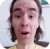FreeKB - Perl Do something range of lines
Perl - Do something range of linesLet's say a file, array, or hash array contains the following.

``````Line 1
Line 2
Line 3
Line 4
Line 5``````

The .. characters are used to do something on a range of lines inside of a loop. In this example, everything between "Line 2" through "Line 4" is printed.

``````my \$file = "/path/to/file.txt";
open(FH, '<', "\$file") or die "cannot open \$file \$! \n";

while (my \$line = <FH>) {
if (\$line =~ /Line 2/ .. \$line =~ /Line 4/) { print \$line; }
}

close FH;``````

This will produce the following result.

``````Line 2
Line 3
Line 4``````

To print everything except Lines 2 through Line 4, use unless instead of if.

``````my \$file = "/path/to/file.txt";
open(FH, '<', "\$file") or die "cannot open \$file \$! \n";

while (my \$line = <FH>) {
unless (\$line =~ /Line 2/ .. \$line =~ /Line 4/) { print \$line; }
}

close FH;``````

This will produce the following result.

``````Line 1
Line 5``````

Let's say the file, array, or hash array contains the following.

``````START
foo
END
START
bar
END``````

And you have the following if statement.

``if (\$line =~ /START/ .. \$line =~ /END/) { print \$line; }``

This will print everything for the first match of "START" to the last match of "END". In this example, the following would be printed.

``````START
foo
END
START
bar
END``````

To limit range to get the first match of "START" to the first match of "END", add the following last if statement.

``````if (\$line =~ /START/ .. \$line =~ /END/) {
print \$line;
last if \$line =~ /END/;
}``````

Now, the following will be returned.

``````START
foo
END``````

We will never share your name or email with anyone. Enter your email if you would like to be notified when we respond to your comment.

Please enter 281b0 in the box below so that we can be sure you are a human.

Web design by yours truely - me, myself, and I   |   jeremy.canfield@freekb.net   |